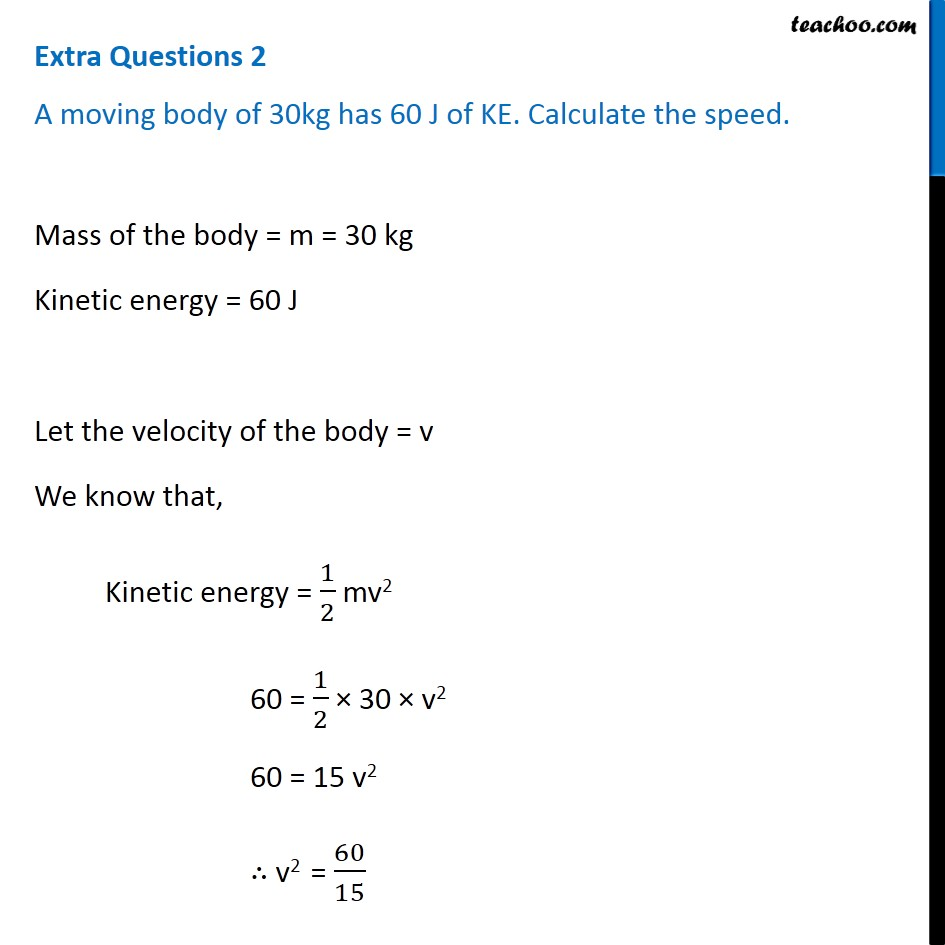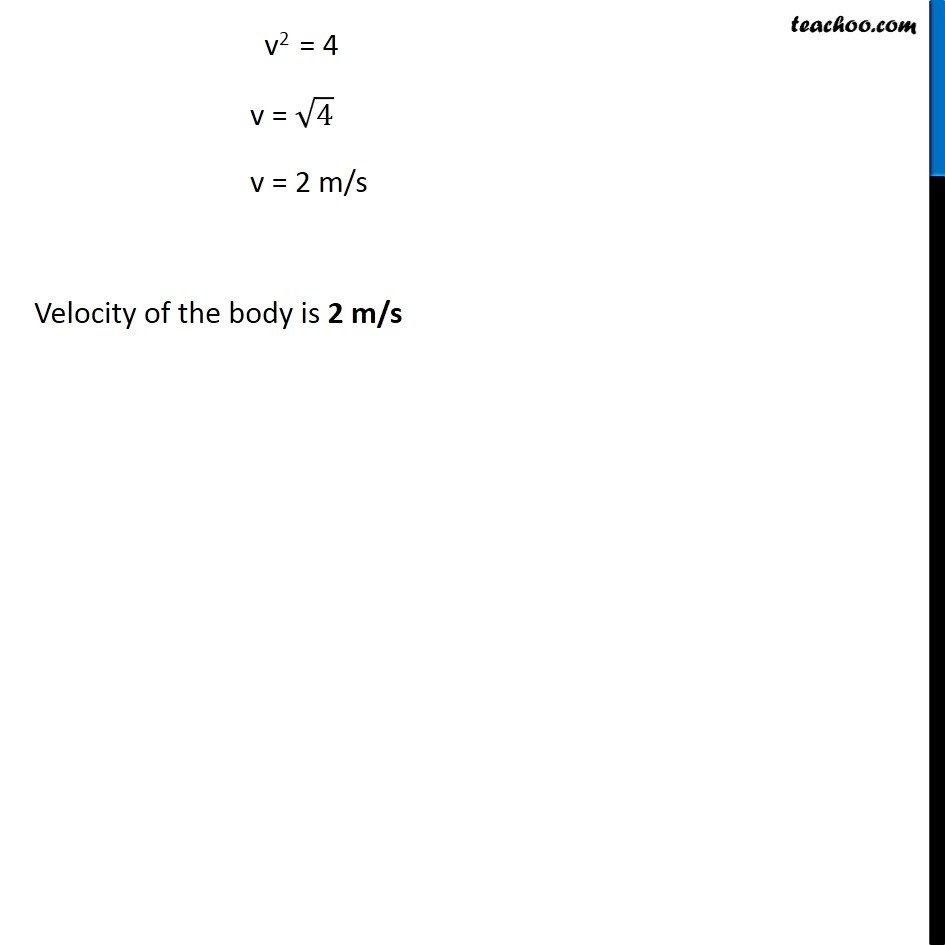Teachoo Questions

Class 9
Chapter 11 Class 9 - Work and EnergyLearn in your speed, with individual attention - Teachoo Maths 1-on-1 Class

### Transcript

Extra Questions 2 A moving body of 30kg has 60 J of KE. Calculate the speed. Mass of the body = m = 30 kg Kinetic energy = 60 J Let the velocity of the body = v We know that, Kinetic energy = 1/2 mv2 60 = 1/2 × 30 × v2 60 = 15 v2 ∴ v2 = 60/15 v2 = 4 v = √4 v = 2 m/s Velocity of the body is 2 m/s